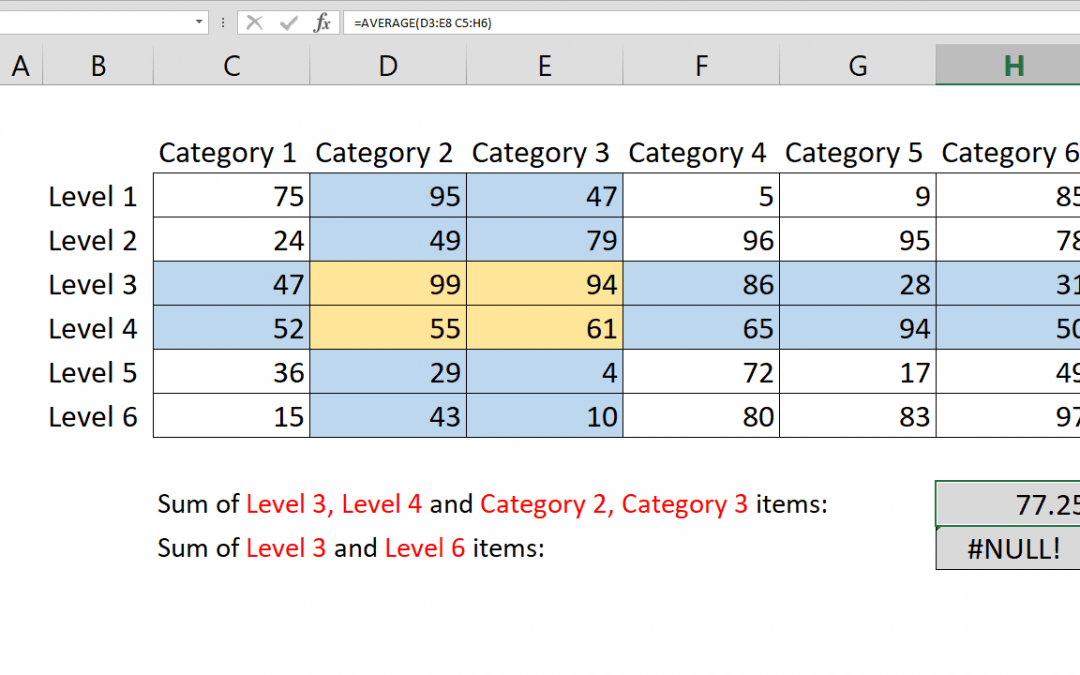## How to calculate AVERAGE with intersecting tables

This article shows how to calculate average with intersecting tables using AVERAGE function and intersect operator, the space character (” “). Syntax =AVERAGE(first range second range) Steps Type =AVERAGE( function Select or type the first range reference...## How to calculate SUM with intersecting tables

This article shows how to calculate sum with intersecting tables using SUM function and intersect operator, the space character (” “). Syntax =SUM(first range second range) Steps Type =SUM( function Select or type the first range reference that includes...